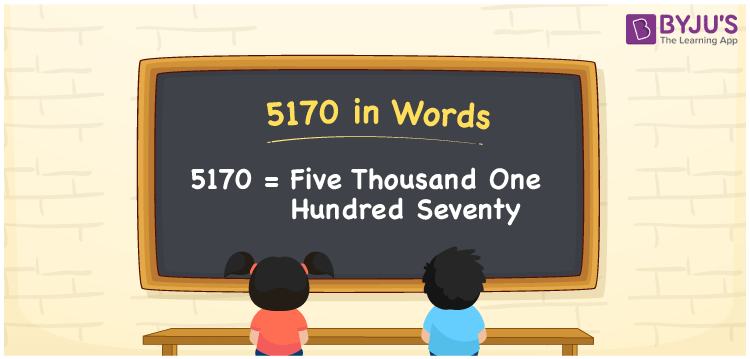# 5170 in Words

We can write the number 5170 in words as Five thousand one hundred seventy. Suppose you spotted an amount of Rs. 5170 credited to your account; then you can say, “My account has been credited with Five thousand one hundred seventy rupees”. In this article, you will learn how to convert the number 5170 into words with the help of a place value chart.

 5170 in words Five thousand one hundred seventy Five thousand one hundred seventy in Numbers 5170

## 5170 in English Words

We generally express numbers in words using the English alphabet. So, we can spell 5170 in English as “Five thousand one hundred seventy”.## How to Write 5170 in Words?

Let’s understand how to write the number 5170 in words using a value chart. The below table displays the place values for all the integers of the number 5170.

 Thousands Hundreds Tens Ones 5 1 7 0

Here, ones = 0, tens = 7, hundreds = 1, and thousands = 5.

Thus, we can expand the above digits with respect to their place values as shown below.

5 × Thousand + 1 × Hundred + 7 × Ten + 0 × One

= 5 × 1000 + 1 × 100 + 7 × 10 + 0 × 1

= 5000 + 100 + 70

= Five thousand + One hundred + Seventy

= Five thousand one hundred seventy

Therefore, 5170 in words = Five thousand one hundred seventy.

5170 is a natural number that precedes 5171 and succeeds 5169.

5170 in words – Five thousand one hundred seventy

Is 5170 an odd number? – No

Is 5170 an even number? – Yes

Is 5170 a prime number? – No

Is 5170 a composite number? – Yes

Is 5170 a perfect square number? – No

Is 5170 a perfect cube number? – No

## Frequently Asked Questions on 5170 in Words

Q1

### How to write 5170 in words?

The number 5170 can be written in words as Five thousand one hundred seventy.
Q2

### How do you write an amount of Rs. 5170 in words on a cheque?

On a cheque, we can express an amount of Rs. 5170 in words as “Five thousand one hundred seventy rupees only.
Q3

### Express the answer in words for 5170 – 170.

5170 – 170 = 5000 Therefore, the value of 5170 – 170, i.e., 5000 in words is Five thousand.